# Mathematical Impressions: Geometry of Spaghetti Code

A sculpture project built entirely with right angles combines math and art in subtle and surprising ways.

Most people find it surprising to learn that this sculpture, Spaghetti Code, is built entirely with right angles. I designed its 180 laser-cut aluminum parts to have slots and tabs that are easily joined together and locked with a pin, so it could be quickly assembled by a large group of people at a “sculpture barn raising.” Laser-cutting allows for affordable and precise fabrication, but the technology requires that the parts join in orthogonal planes. In order to design the sculpture, I first needed to explore an interesting problem in applied mathematics: to select a set of planes in which many pairs are orthogonal. Furthermore, I like the visual and structural qualities that symmetry imparts, so I wanted the entire design to have icosahedral symmetry.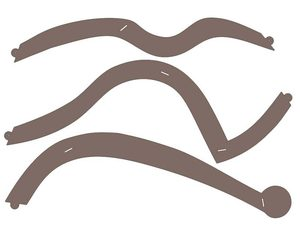This project combines math and art in subtle ways. I ended up creating three shapes of parts, sixty of each, in which the curves largely follow my artistic whims. But the relative positions of the tabs and slots are tightly constrained by the geometry so that the mortise and tenon joints meet orthogonally.First, keep in mind that the issue here is the angle between planes in space, not the angle between lines in a plane. Euclid showed that an equilateral triangle in the plane must have three 60-degree angles, but that is not the concern here. It is possible to construct a physical equilateral triangle in space using three identical planar components that meet at 90-degree angles to each other. This can be visualized by cutting off strips near the corners of a cube.By modifying the cuts in the faces of a cube, it is possible to have curved components take arbitrary meandering paths, yet still join orthogonally. All that is necessary is that the curves terminate at consistent points on the lines where the orthogonal planes meet. This example uses an arbitrary curve to show that it is easy to produce 3-fold rotational symmetry using a single shape of planar component and only orthogonal joints.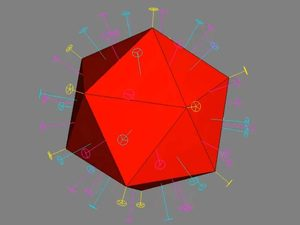Spaghetti Code has icosahedral symmetry, i.e., it has the same rotational axes as an icosahedron. There are six 5-fold axes, ten 3-fold axes, and fifteen 2-fold axes. Together, these 31 axes characterize the geometry of the icosahedral symmetry group. Observe that the triangular face planes of the icosahedron are each orthogonal to one of the 3-fold axes and all lie the same distance from the center.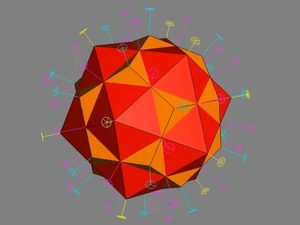If we abstract away from the polyhedron and just consider the arrangement of these axes in space, we find these same thirty-one axes are also the symmetry elements of the dodecahedron. This image of an icosahedron and dodecahedron intersecting each other “in dual position” shows they have the same set of rotational axes. Each polyhedron has vertices above the face centers of the other. Each edge of either polyhedron crosses an edge of the other polyhedron orthogonally. See that the 5-fold axes go through the vertices of the icosahedron and the faces of the dodecahedron. Reciprocally, the 3-fold axes go through the vertices of the dodecahedron and the faces of the icosahedron.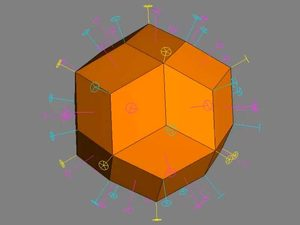My starting point for this sculpture is just the set of symmetry axes. I can then construct planes orthogonal to any type of axis, at a chosen distance from the origin. Taking the intersection of the inner half-spaces defined by those planes gives a polyhedron that is useful for visualizing the planes. If I choose the 3-fold or 5-fold axes, this construction gives the icosahedron or dodecahedron, respectively. If instead I choose the 2-fold axes, intersecting the half-spaces of the orthogonal planes gives the rhombic triacontahedron. It comprises thirty rhombi, as illustrated here.For Spaghetti Code, I chose the thirty planes of the 2-fold axes plus the twelve planes of the 5-fold axes—forty-two planes in all. These can be visualized with the help of a truncated rhombic triacontahedron, shown here. But my canvas for creating flat sculptural components can be the entire plane, including the outer regions away from the polyhedron. Note that the ratio of the distance from the origin to the two families of planes is a free parameter, which I can change for artistic purposes without affecting the angle between planes. Also observe that this polyhedron should not be confused with the simpler, well-known truncated icosahedron, which is most familiar as a soccer ball.I chose these forty-two planes because of the many orthogonal pairs among them. They can be visualized and understood via the relationship between the cube and the dodecahedron. In Book 13 of The Elements, Euclid starts with a cube and adds a “roof” to each square in a way that provably results in twelve regular pentagons. Since we already know that regular dodecahedra exist, it is easier for us to go in the opposite direction from Euclid—to inscribe cubes in the dodecahedron. Choose eight dodecahedron vertices that appear to outline a cube, and connect them as shown above. To prove this really is a cube, first observe that each apparent cube edge is a diagonal of a pentagon. Since the pentagons of a regular dodecahedron are regular and equal, their diagonals are all equal, so these twelve segments that look like a cube’s edges are equal. Then to see these are truly squares, and not just rhombi or nonplanar quadrilaterals, look at the mirror planes of the dodecahedron. Our putative squares are mirror symmetric in two orthogonal mirror planes, so they must be rectangles. Combining the facts that they have four equal edges and that they are rectangles proves they are squares. So what appears to be a cube really is a cube.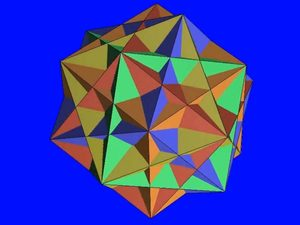By rotating the dodecahedron and inscribed cube together about a 5-fold axis of the dodecahedron, we can construct five such cubes within a single dodecahedron. For clarity in the image above, each cube is a different color and the outer dodecahedron is omitted. Now ask: what is the shape of the volume interior to all five cubes? That turns out to be the rhombic triacontahedron, because it is the intersection of the half-spaces defined by the thirty planes of the cubes’ faces, which are orthogonal to the 2-fold axes. Each of the five cubes has twelve edges, so among these thirty planes, there are sixty orthogonal pairs. But there is no path of orthogonally connectable planes that connects one cube with any of the other four cubes. So a sculpture using only these planes and the orthogonal connections they allow would separate into five separate components. However, adding the dodecahedron face planes provides additional orthogonalities, resulting in a fully connected graph of orthogonal connections. To understand why, one can go back to the cube in the dodecahedron and carefully observe that each cube face is orthogonal to four pentagons. So there are 120 orthogonal pairs of planes of this sort. When we add these to the 60 orthogonal pairs just among the cube faces we obtain 180 pairs in total, allowing sufficiently many connections for me to have all the components of the sculpture hold together.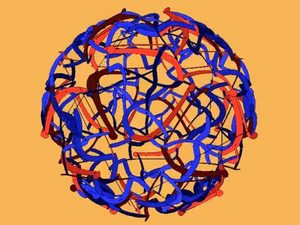These mathematical ideas provide families of planes with many perpendicular pairs. I can adjust the distance from the origin to the planes independently for each family of axes and then solve for the lines where pairs of planes intersect. Positioning the tabs and slots on these lines in space ensures that the parts will meet other parts properly. Whatever I draw in any plane can be replicated to its entire orbit of planes under the set of rotations of the icosahedral symmetry group. I have coded this mathematical framework into custom, interactive software that allows me to draw interesting curves in those planes and create a visually engaging 3D form. In this screen shot, components in the rhombic triacontahedron planes are shown blue and components in the dodecahedron planes are shown red. The underlying geometry subtly guides the design, yet leaves me free to explore within these constraints. This is where the math ends and the art begins.

More videos from the Mathematical Impressions series.